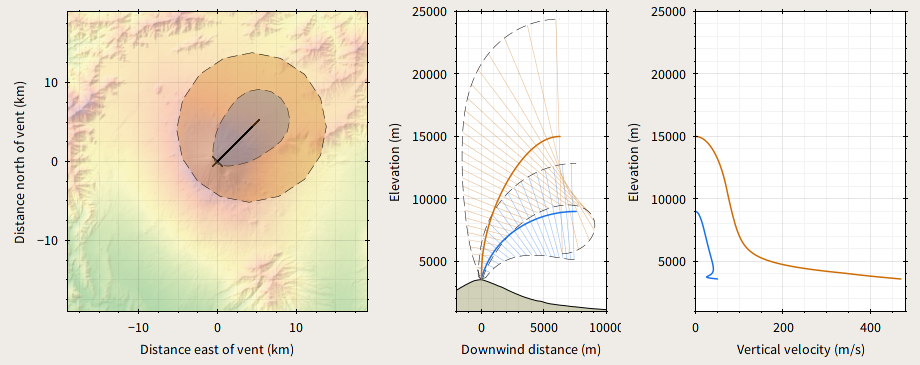PlumeRise
Documentation
Inferring source mass flux from plume top height

PlumeRise can be used to estimate the source mass flux from an observation of the rise height of a volcanic plume by adjusting the source velocity. This 'inverse' calculation is performed by selecting the 'Infer source flux from observed plume rise height' radio button in the 'Parameters' tab and entering a target height in the 'Rise height (m)' text box.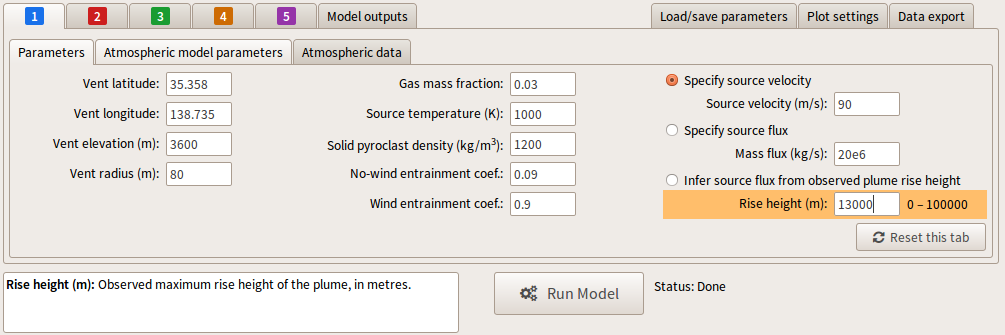The inversion calculation first performs a series of model runs across the range of allowed source velocities (1 – 500 m/s) to assess the likelihood that the target height can be achieved. If the target height lies within the range determined by series of model runs then an iterate refinement of the source velocity is performed in order to match the calculated plume height to the target value. If the target height cannot be achieved then a status message reports the likely range of plume heights that could be achieved.

Example

A plume rising from a vent at an elevation of 3600 m is observed to rise to a height of 9000 m above sea level. The vent radius is estimated to be 80 m, the source temperature is estimated to be 1200 K, and the plume is ash-rich with an estimated solids mass fraction of 97%. A US Standard Atmosphere is used to describe the atmosphere, with a stratospheric wind speed of 50 m/s.

In order to assess the possibility that the plume height can match the target value with the source estimates above, two calculations with specified source velocities (one at a relatively low value 20 m/s, the other at a higher value of 300 m/s) can be performed by assigning parameters in tabs 1 and 2. The settings used are shown below.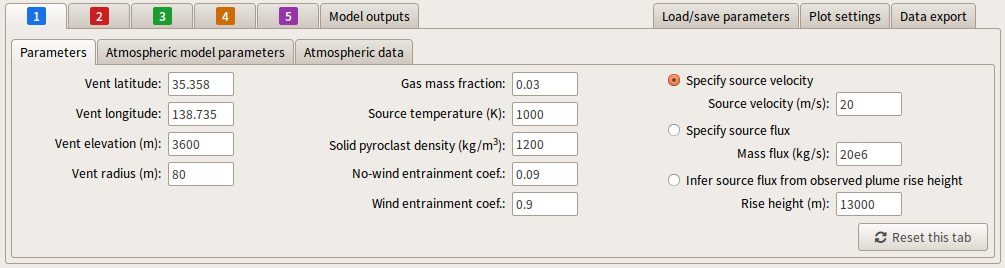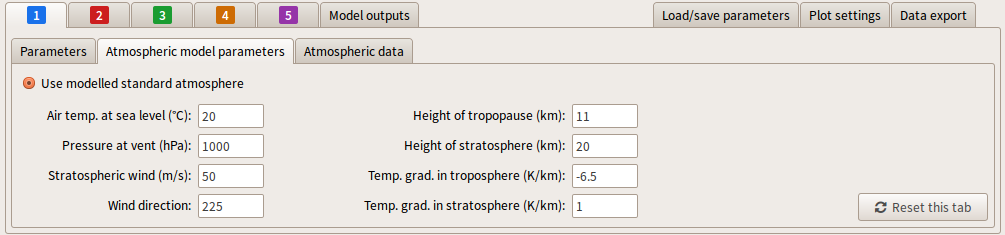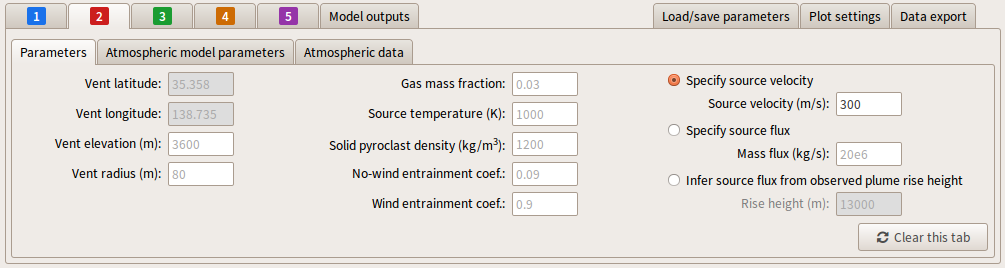Running the calculation we obtain two solutions. As shown in the 'Model outputs', the parameter set 1 produces a plume rising to an altitude of 7527 m asl and parameter set 2 gives a rise height of 12724 m asl.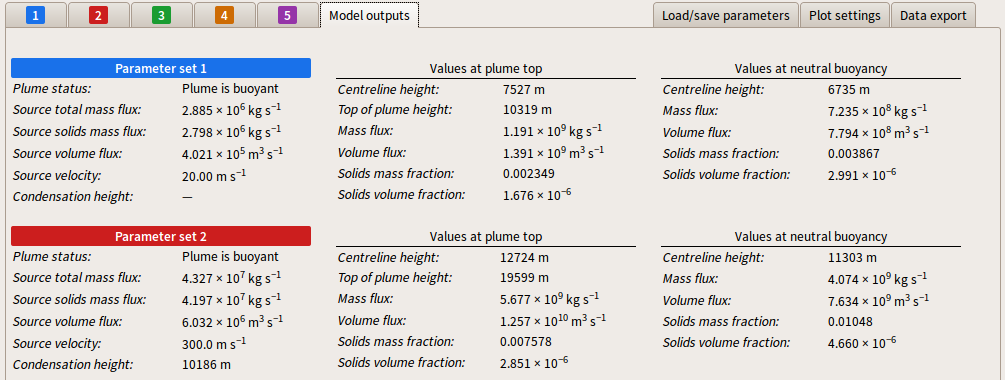The preliminary calculations suggest a source flux can be found to match the predicted plume top height to the target value of 9000 m. In parameter set 3 the target plume top height is entered in the 'Rise height (m)' text box.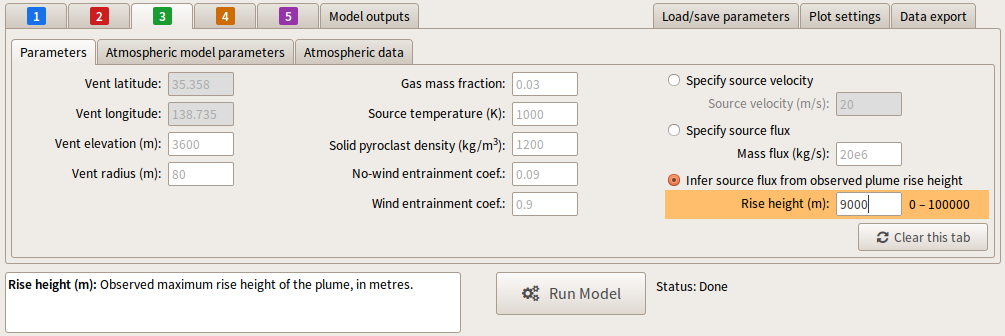Running the model, PlumeRise is able to determine a source mass flux that produces a plume rising to the required height of 9000 m asl.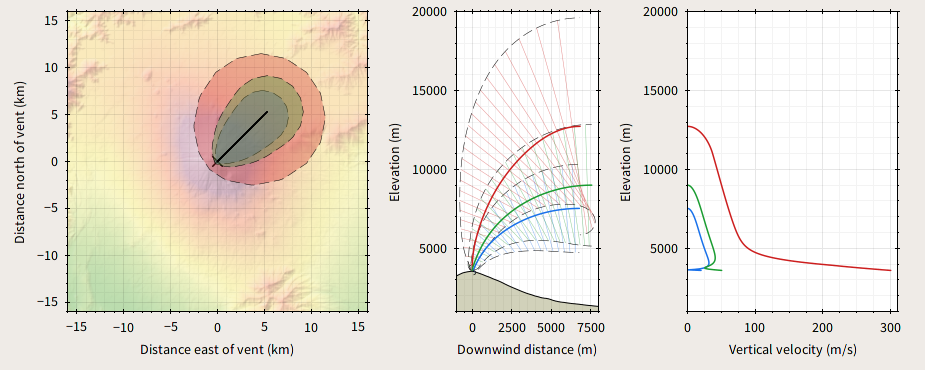The Model Outputs for parameter set 3 shows the calculated source mass flux and the corresponding source velocity.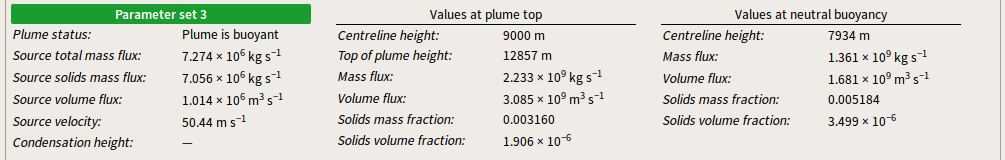If we instead seek a plume rising to 15 km asl (for example by entering this height into the 'Rise height (m):' box in parameter set 4), PlumeRise is unable to find a solution. On running the model, a status message is returned suggesting the plume height cannot reach above 14033 m asl for the parameters used.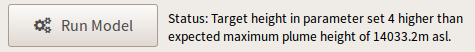Therefore, in order to reach an altitude of 15 km asl, some of the other source conditions must be altered. For example, if the vent radius is increased to 100 m a solution with a plume top height of 15 km asl can be found. The figure below shows a comparison of 9 km plume and the 15 km plume. (Note the settings to determine the 9 km high plume have been moved into parameter set 1.)